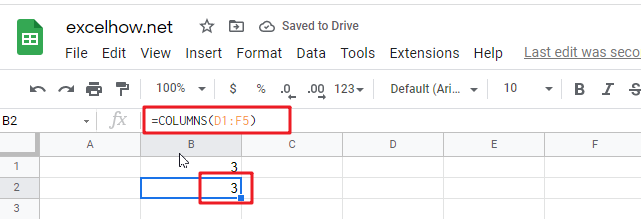## Calculate The Average Of The Last 3, 5, Or N Numeric Values In Google Sheets

As an Google sheets user, you might have come across a task in which you need to calculate the average values of the last 2 numeric values, and you might have done this task manually but suppose if the last values you want to calculate the average exceeds to 3, 5 or N numeric values then what would you do? If you would tend to do this task manually, then it would be your foolish decision because doing these kinds of lengthy tasks manually is very hard, and when it comes to N numeric values, then It would not be wrong to say that this is near to impossible to do it manually.

But don’t worry about it because after carefully reading this article, you can easily calculate the average values of the last 3, 5, and most important N numeric values within seconds.

So without any further delay, let’s dive into it;

## Calculate The Average Of The Last 3 Values In Google Sheets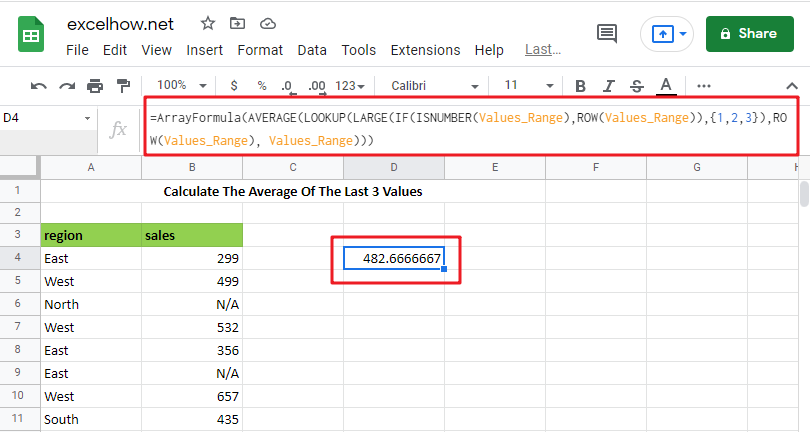The General Formula is as below based on the AVERAGE function, LOOKUP function, LARGE function and IF function:

`=AVERAGE(LOOKUP(LARGE(IF(ISNUMBER(Values_Range),ROW(Values_Range)),{1,2,3}),ROW(Values_Range), Values_Range))`

Note: this above is an array formula, you need to press “CTRL+SHIFT+ENTER” short cuts to make it as array formula.

## Let’s See How This Formula Works

You can use an array formula for getting the average of the last 3 values in the range. The formula in E3 in the example is as follows:

`=AVERAGE(LOOKUP(LARGE(IF(ISNUMBER(Values_Range),ROW(Values_Range)),{1,2,3}), ROW(Values_Range), Values_Range))`Here “ Values_Range ” refers to the designated name range B4:B11.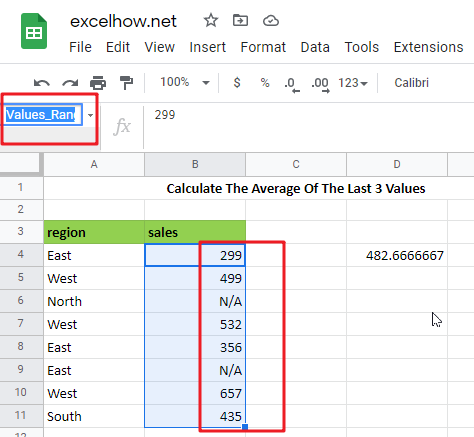This is an array formula, so input it using control + Shift + enter.

Because the AVERAGE function computes an average of numbers supplied in an array, practically all of the effort in this formula is to construct an array of the latest three numeric values in a range.

=IF(ISNUMBER(Values_Range),ROW(Values_Range))

The IF function in the formula is used to “filter” numeric numbers from the inside out.

Because the ISNUMBER function returns TRUE for numeric values and FALSE for other values (including blanks), and the ROW function produces row numbers, the outcome of this operation is an array of row numbers corresponding to numeric entries:

`={4;5;FALSE;7;8;FALSE;10;11}`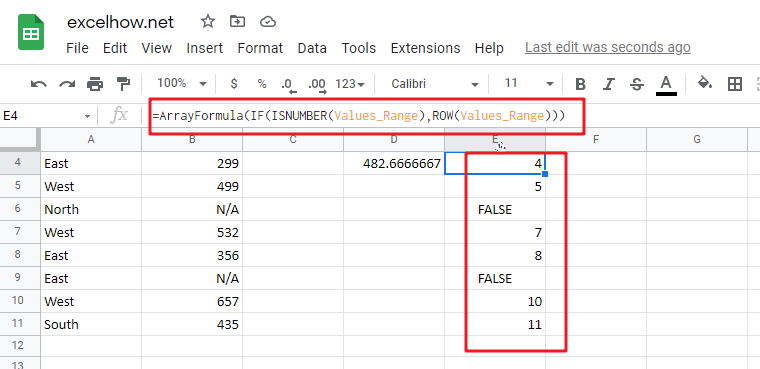=LARGE({4;5;FALSE;7;8;FALSE;10;11},{1,2,3})

The above array is sent to the LARGE function, using the array constants 1,2,3 for k. LARGE ignores FALSE values and returns an array with the greatest three integers, which correspond to the last three rows with numeric values:

`={11,10,8}`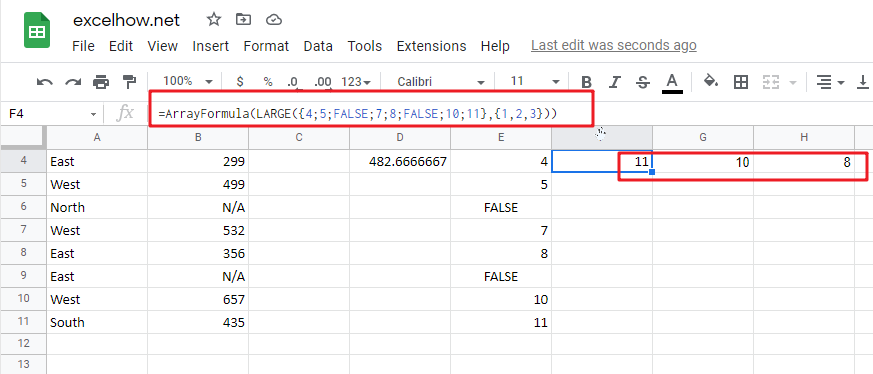=LOOKUP({11,10,8}, ROW(Values_Range), Values_Range))

The above array is used as the lookup value in the LOOKUP function. The ROW function provides the lookup array, and the return array is the named range “ Values_Range “:

`={435,657,356}`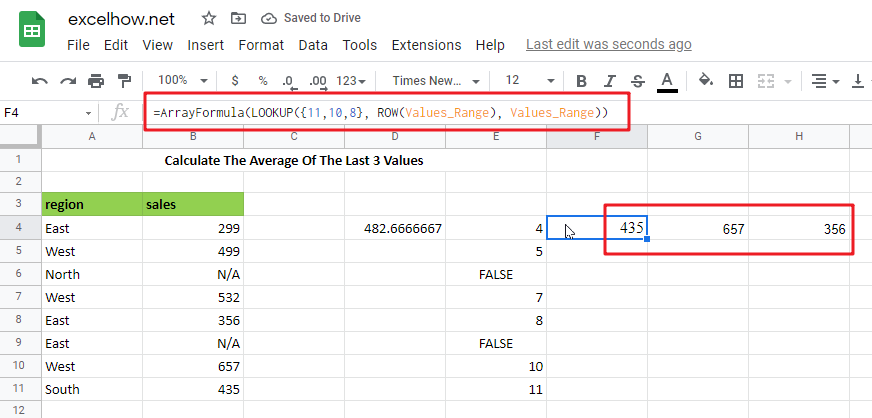The array of similar values in “ Values_Range ” returned by LOOKUP is then put into AVERAGE:

`=AVERAGE({435,657,356})`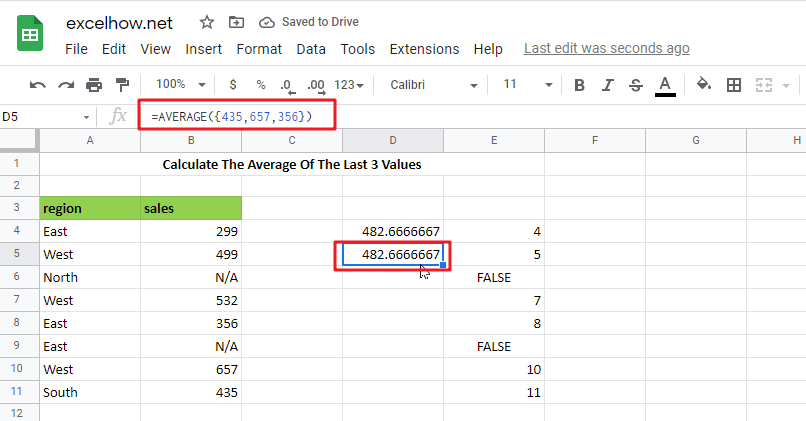## Calculate The Average Of The Last 5 Values IN Google Sheets

Please use the following array formula, which would assist you in calculating the last 5 values in Google Sheets:

In a blank cell, type the following formula:

`=IF(COUNT(B:B),AVERAGE(INDEX(B:B,LARGE(IF(ISNUMBER(B1: B10000),ROW(B1: B10000)),MIN(5,COUNT(B1: B10000)))):B10000),"no data found")`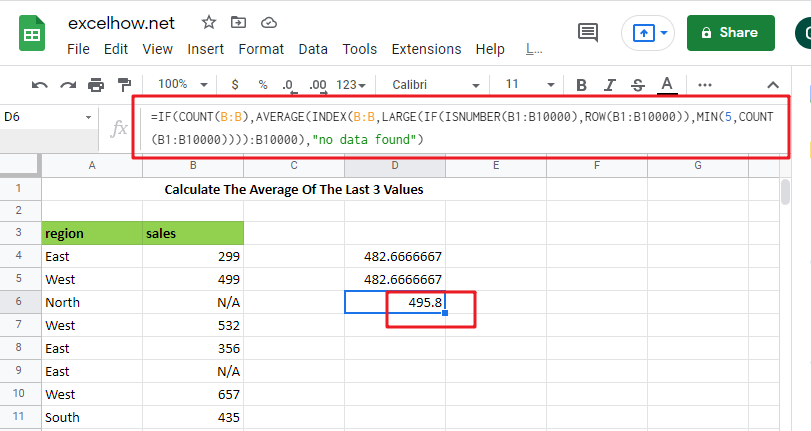Note: B:B is the column that holds the data you used, B1: B10000 is a dynamic range that you may stretch as long as you need, and the number 5 represents the latest n values, and then press Ctrl + Shift + Enter to make the formula as array formula to obtain the average of the last 5 numbers. See the following screenshot:

And now, when you enter new numbers underneath the old data, the average is updated as well, as seen in the screenshot: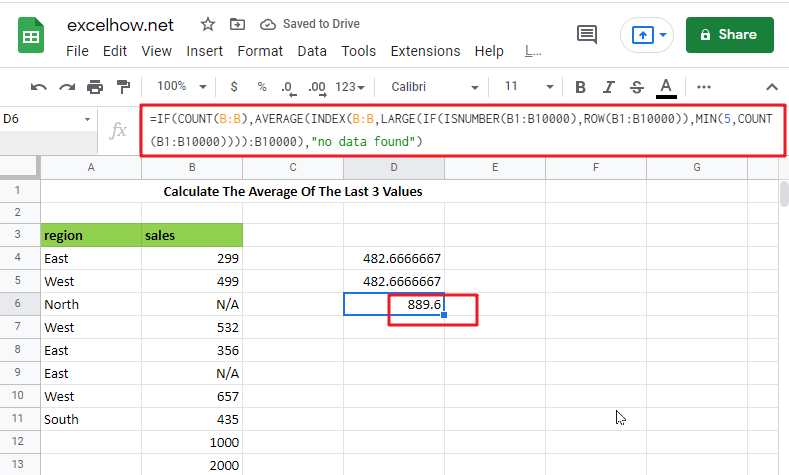### Related Functions

The Google Sheets ROW function returns the row number of a cell reference.The ROW function is a build-in function in Google Sheets and it is categorized as a Lookup and Reference Function.The syntax of the ROW function is as below:= ROW ([reference])….
The Google Sheets IF function perform a logical test to return one value if the condition is TRUE and return another value if the condition is FALSE. The IF function is a build-in function in Google Sheets and it is categorized as a Logical Function.The syntax of the IF function is as below:= IF (condition, [true_value],
The Google Sheets ISNUMBER function returns TRUE if the value in a cell is a numeric value, otherwise it will return FALSE.The syntax of the ISNUMBER function is as below:= ISNUMBER (value)…
The Google Sheets LOOKUP function will search a value in a vector or array.The syntax of the LOOKUP function is as below:= LOOKUP (lookup_value, lookup_vector, [result_vector])…
The Google Sheets AVERAGE function returns the average of the numbers that you provided.The syntax of the AVERAGE function is as below:=AVERAGE (number1,[number2],…)….
The Google Sheets LARGE function returns the largest numeric value from the numbers that you provided. Or returns the largest value in the array. The syntax of the LARGE function is as below:= LARGE (array,nth)…
The Google Sheets COUNT function counts the number of cells that contain numbers, and counts numbers within the list of arguments. It returns a numeric value that indicate the number of cells that contain numbers in a range…
The Google Sheets INDEX function returns a value from a table based on the index (row number and column number)The INDEX function is a build-in function in Google Sheets and it is categorized as a Lookup and Reference Function.The syntax of the INDEX function is as below:= INDEX (array, row_num,[column_num])…
The Google Sheets MIN function returns the smallest numeric value from the numbers that you provided. Or returns the smallest value in the array.The MIN function is a build-in function in Google Sheets and it is categorized as a Statistical Function.The syntax of the MIN function is as below:= MIN(num1,[num2,…numn])….
The Google Sheets ROW function returns the row number of a cell reference.The ROW function is a build-in function in Google Sheets and it is categorized as a Lookup and Reference Function.The syntax of the ROW function is as below:= ROW ([reference])….
The Google Sheets SUBTOTAL function returns the subtotal of the numbers in a list or database. The syntax of the SUBTOTAL function is as below:= SUBTOTAL (function_num, ref1, [ref2])….

This post will guide you how to use Google Sheets ROWS function with syntax and examples.

## Description

The Google Sheets ROWS function counts the number of rows in a cell reference.

The ROWS function can be used to return the number of rows in a specified range in google sheets. The purpose of this function is to get the number of rows in an array or cell reference and It’s returned value is a number of rows.

The ROWS function is a build-in function in Google Sheets and it is categorized as a LOOKUP function.

## Syntax

The syntax of the ROWS function is as below:

= ROWS(array)

Where the ROWS function arguments is:

• array-This is an required argument. An array, or A reference to a range of cells.

Note:

• ROWS just takes one argument, it can be a range or a array.
• Array can be a range or a cell reference
• If you want to count columns, you can use the COLUMNS function.
• If you want to get row numbers, you can use the ROW function.

## Google Sheets ROWS Function Examples

The below examples will show you how to use google sheets ROWS Function to return the number of rows in a range of cells.

#1 To get the number of rows in the cell range “A1:C4”, just using the following ROWS formula:

=ROWS(A1:C4)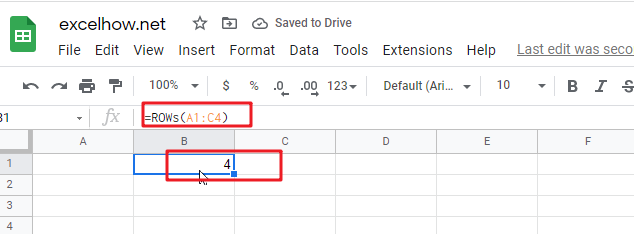This post will guide you how to use Google Sheets ROW function with syntax and examples.

## Description

The Google Sheets ROW function returns the row number of a cell reference.

The ROW function can be used return the row number of a specified cell in google sheets. The purpose of this function is to get the row number of a reference and It’s returned value is a number representing the row.

The ROW function is a build-in function in Google Sheets and it is categorized as a LOOKUP function.

## Syntax

The syntax of the ROW function is as below:

= ROW ([reference])

Where the ROW function argument is:

• Reference – This is an Optional argument. the cell or range of cells reference that you want to get the row number.

Note:

• If the reference is omitted, the ROW function will return the row number of the current cell.
• Reference can be a single cell address or a range of cells.
• Reference can not include multiple cell references.
• If you want to get column number, you can use the COLUMN function.
• If you want to count rows, you can use the ROWS function.

## Google Sheets ROW Function Examples

The below examples will show you how to use google sheets ROW Function to return the row number of a cell reference.
#1 To get the row number of “B5” cell in B1 Cell, just using the following ROW formula:

` =ROW(B5)`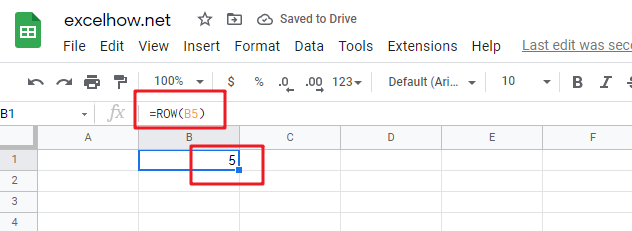#2 To get the row number of the current cell, just using below formula:

`=ROW()`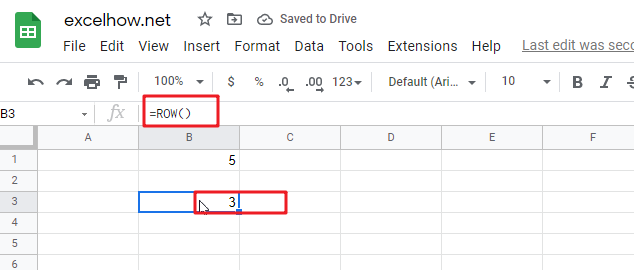#3 get the first row number of range in excel

You can get the first row number in a range with an formula based on the ROW function as follows:

```=ROW(Range)
=MIN(ROW(Range))```

The ROW function will only display the first row number.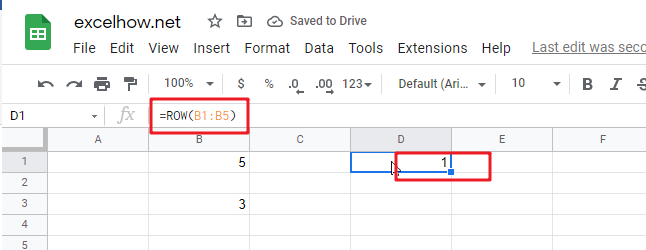This post will guide you how to use Google Sheets COLUMNS function with syntax and examples.

## Description

The Google Sheets COLUMNS function returns the number of columns in an Array or a reference. For example, COLUMNS(B1:D4) returns 3, since the range B1:D4 contains 3 columns.

The COLUMNS function can be used to get the count of columns in a given cell range in google sheets. The purpose of this function is to get the number of columns in an array range or cell reference and the returned value is a number that representing the COLUMNS.

The COLUMNS function is a build-in function in Google Sheets and it is categorized as a Lookup function.

## Syntax

The syntax of the COLUMNS function is as below:

=COLUMNS (array)

Where the COLUMNS function arguments are:

• Array -This is a required argument. A reference to a cell or a range of cells.

Notes:

• Array can be a cell range or a reference that containing a group of cells
• If you want to get column numbers, just see also COLUMN function.
• If you want to get row numbers, just see also ROW function.
• If you want to get the number of rows in given array or cell range, just see also ROWs function.

## Google Sheets COLUMNS Function Examples

The below examples will show you how to use google sheets COLUMNS to return the number of columns in a given range.

#1 To get the number of columns in the reference D1:F5, just using the following excel formula:

`=COLUMNS(D1:F5)`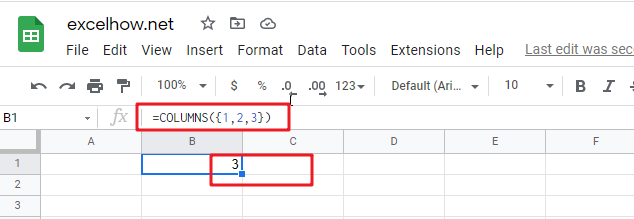#2 Using COLUMNS function to get a column count for a given array constant “{1,2,3}”, just using the following formula:

`=COLUMNS({1,2,3})`